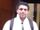## Multiplying a variable with its corresponding frequency

Hi all,

I am a sas beginner and I wanted to do the following. I have a variable "int" that has integers and when I run proc freq on it I get how many observations are associated with each variable. For example:

int              Frequency

0.17           1123

0.92           156

1                 2300

1.42            2152

1.67            635

I want to create a new variable that multiplies int*frequency. I then want a total sum of this new variable. Any help would be appreciated, thank you!

1 ACCEPTED SOLUTION

Accepted Solutions

## Re: Multiplying a variable with its corresponding frequency

If you step away from PROC FREQ for a moment, you can get this from your original data set directly:

proc summary data=original nway;

class int;

var int;

output out=want (keep=int total) sum=total;

run;

6 REPLIES 6novinosrin
Tourmaline | Level 20

## Re: Multiplying a variable with its corresponding frequency

``````data have;
input int              Frequency;
cards;
0.17           1123
0.92           156
1                 2300
1.42            2152
1.67            635
;

proc sql;
create table want as
select *,int*frequency as multi, sum(calculated multi) as sum
from have;
quit;``````

## Re: Multiplying a variable with its corresponding frequency

``````proc summary data=have;
var int;
weight frequency;
output out=want sum=;
run;``````
--
Paige Miller

## Re: Multiplying a variable with its corresponding frequency

Hi all, thank you for the help! It seems you are suggesting I make a new data set where I manually enter the "int" and "frequency" values. I have hundred of  "int" values however so it would take me a while to run proc freq on int then create a dataset using the frequency proc freq generated for me. is there another way to do it that doesn't involve me manually creating a new dataset?

thanks!

## Re: Multiplying a variable with its corresponding frequency

@Ethid wrote:

Hi all, thank you for the help! It seems you are suggesting I make a new data set where I manually enter the "int" and "frequency" values.

This is entirely a different issue. Nowhere did I suggest you make a new data set. You can do this with your existing SAS data set.

I have hundred of  "int" values however so it would take me a while to run proc freq on int then create a dataset using the frequency proc freq generated for me.

This is not correct, PROC FREQ ought to run quickly. It won't multiply the values as you requested.

is there another way to do it that doesn't involve me manually creating a new dataset?

You don't have to make a new dataset.

--
Paige Miller

## Re: Multiplying a variable with its corresponding frequency

If you step away from PROC FREQ for a moment, you can get this from your original data set directly:

proc summary data=original nway;

class int;

var int;

output out=want (keep=int total) sum=total;

run;

## Re: Multiplying a variable with its corresponding frequency

Hello,

You can add this to the end of your Freq table statement.

tables int / out=xx;

data new_result;

set xx;

new_data=int*count;

run;

Discussion stats
• 6 replies
• 1563 views
• 4 likes
• 5 in conversation